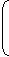# Aptitude :: Compound Interest

Exercise : Compound Interest - Formulas
1. Let Principal = P, Rate = R% per annum, Time = n years.

2. When interest is compound Annually:

 Amount = P1 + Rn 100

3. When interest is compounded Half-yearly:

 Amount = P1 + (R/2)2n 100

4. When interest is compounded Quarterly:

 Amount = P1 + (R/4)4n 100

5. When interest is compounded Annually but time is in fraction, say 3years.

 Amount = P1 + R3 x1 +R100 100

6. When Rates are different for different years, say R1%, R2%, R3% for 1st, 2nd and 3rd year respectively.

 Then, Amount = P1 + R11 + R21 + R3. 100 100 100

7. Present worth of Rs. x due n years hence is given by:

Present Worth = x1 + Rn 100